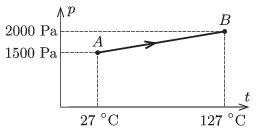Mathematical and Physical Journal
for High Schools
Issued by the MATFUND Foundation
 Already signed up? New to KöMaL?

#Problem P. 4632. (April 2014)

P. 4632. A sample of low pressure gas of given mass, is taken from state $\displaystyle A$ to state $\displaystyle B$, along the process $\displaystyle AB$ as shown in the figure. How much work is done?(4 pont)

Deadline expired on May 12, 2014.

Sorry, the solution is available only in Hungarian. Google translation

Megoldásvázlat. A folyamat izochor, nem végzünk munkát.

### Statistics:

 64 students sent a solution. 4 points: 59 students. 3 points: 2 students. 2 points: 2 students. 0 point: 1 student.

Problems in Physics of KöMaL, April 2014